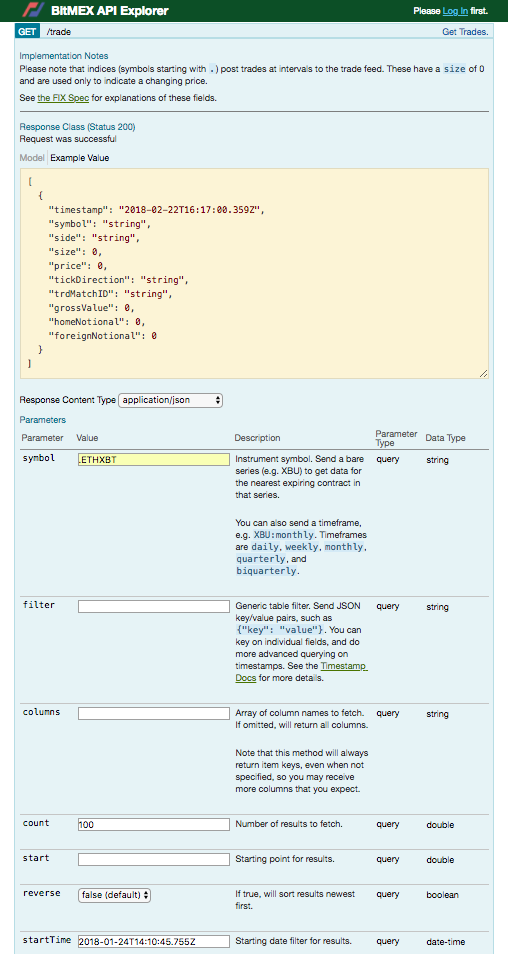We do not store the mark price on our site, however it is possible to recreate the mark price at the time of your liquidation, using the REST API, in order to verify if a liquidation was correctly processed by the liquidation engine.

Using the formula presented in our references and our API explorer you can easily calculate the mark price at the exact time of your liquidation if you follow the steps below.

This is the formulas used for calculating the mark price:

• % Fair Basis = (Impact Mid Price / Index Price - 1) / (Time To Expiry / 365)
• Fair Value = Index Price * % Fair Basis * (Time to Expiry / 365)
• Fair Price = Index Price + Fair Value

You can pull the data from the REST API, using either the direct links or the API explorer, the respective end points for the API explorer are:

*Please note that if /Quote is disabled, you can find /quote data dumps via public.bitmex.com

To Begin (the example used is ETHH18)

There are a few details which you must ensure that you include if you would like the correct results to be printed by the REST API, below is an image of BitMEX's API Explorer.1) The most common mistake is inputting an incorrect startTime and endTime, both dates need to be in JSON format.

Provided in the yellow example box: 2018-02-22T15:49:06.628Z = 22 February 2018 at 15:49:06 AM UTC, where the 06 is seconds & the .628Z is the milliseconds.

You need to ensure that your startTime and endTime interval include the timestamp of your liquidation.

Part 1: .ETHXBT Index

We find the following API link:

With the following response body (the data you need):

``````[
{
"timestamp": "2018-01-24T14:11:00.000Z",
"symbol": ".ETHXBT",
"size": 0,
"price": 0.0925,
"tickDirection": "ZeroPlusTick",
"trdMatchID": "00000000-0000-0000-0000-000000000000",
"grossValue": null,
"homeNotional": null,
"foreignNotional": null
}
]``````

Part 2: Impact Mid PriceWe find the following API link:

https://www.bitmex.com/api/v1/quote?symbol=ETHH18&count=100&reverse=false&startTime=2018-01-24T14%3A09%3A00.000Z&endTime=2018-01-24T14%3A11%3A00.000Z

{

"timestamp": "2018-01-24T14:10:45.755Z",

"symbol": "ETHH18",

"bidSize": 19,

"bidPrice": 0.09136,

Thereafter you need to calculate the mid price, which is the average of both the bidPrice and the askPrice.

Historical mid price: (0.09136+0.09173)/2 = 0.091545

Please note this example assumes the quantity on the top of orderbook exceeds the 'Impact Margin Notional' for the instrument, therefore the simple 'Mid Price' calculated here is equal to the 'Impact Mid Price'. If market making conditions require, you may need to sum up multiple levels of the order book to reproduce the Impact Bid/Impact Ask - the process for doing this is beyond the scope of this document, please contact support@bitmex.com if you require more information on this topic.

*Please note that if /Quote is disabled, you can find /quote data dumps via public.bitmex.com

Part 3: Time until expiry

Check the contract page for the expiry date

 Expiry Date Mar 30, 2018, 2:00:00 PM

Mark price date in question: 24th of Jan

Time to expiry: 65 days

Now that you have the respective data you can calculate the exact mark price at the time-stamp of your liquidation.

We recall the formulas used for calculating the mark price:

1. % Fair Basis = (Impact Mid Price / Index Price - 1) / (Time To Expiry / 365)
2. Fair Value = Index Price * % Fair Basis * (Time to Expiry / 365)
3. Fair Price = Index Price + Fair Value

From the data derived we know the following:

Impact Mid price = 0.091545

Index price = 0.0925

Time to expiry = 65

1) (0.091545/0.0925 -1)/(65/365)* 100 = - 5.79750519751%

2) 0.0925*(-0.05797505197)*(65/365) = -0.00095499999

Fair mark price = 0.0925 -0.00095499999

0.09154500001

Therefore we can conclude that the liquidation was valid if the fair price you calculate and the mark price on your email match after rounding and that the liquidation engine properly liquidated your position in accordance with the liquidation engines parameters as set by the formulas.

If you need any further clarification, please don't hesitate to contact support.